# Line Chart with Confidence Interval in Python

## Using Matplotlib, Seaborn, Altair and Plotly to create a line chart with a confidence interval

It's common when working with timeseries, plotting A/B testing results, or conducting other types of analysis to want to plot a line (often the mean of your data over time) and a confidence interval to represent uncertainty in the sample data.

Below we show how to create line charts with confidence intervals using a range of plotting libraries, including interactive versions with Altair and Plotly.

## Imports & Setup

Show Code
``````import altair as alt
from matplotlib import pyplot as plt
from matplotlib_inline.backend_inline import set_matplotlib_formats
import numpy as np
import pandas as pd
import plotly.graph_objects as go
import seaborn as sns

set_matplotlib_formats('retina')

# Create our new styles dictionary for use in rcParams.
dpi = 144
mpl_styles = {
'figure.figsize': (6, 4),
# Up the default resolution for figures.
'figure.dpi': dpi,
'savefig.dpi': dpi,
}

sns.set_theme(context='paper')
plt.rcParams.update(mpl_styles)
``````

## Data

Below we grab some timeseries data from Seaborn's built-in datasets. We keep two versions of the data:

• `df`: "Raw" data with multiple observations per date.
• `df_grouped`: Aggregated data with one row per date.
``````df = sns.load_dataset('taxis')
``````
pickup dropoff passengers distance fare tip tolls total color payment pickup_zone dropoff_zone pickup_borough dropoff_borough pickup_date
0 2019-03-23 20:21:09 2019-03-23 20:27:24 1 1.60 7.0 2.15 0.0 12.95 yellow credit card Lenox Hill West UN/Turtle Bay South Manhattan Manhattan 2019-03-23
1 2019-03-04 16:11:55 2019-03-04 16:19:00 1 0.79 5.0 0.00 0.0 9.30 yellow cash Upper West Side South Upper West Side South Manhattan Manhattan 2019-03-04
2 2019-03-27 17:53:01 2019-03-27 18:00:25 1 1.37 7.5 2.36 0.0 14.16 yellow credit card Alphabet City West Village Manhattan Manhattan 2019-03-27
3 2019-03-10 01:23:59 2019-03-10 01:49:51 1 7.70 27.0 6.15 0.0 36.95 yellow credit card Hudson Sq Yorkville West Manhattan Manhattan 2019-03-10
4 2019-03-30 13:27:42 2019-03-30 13:37:14 3 2.16 9.0 1.10 0.0 13.40 yellow credit card Midtown East Yorkville West Manhattan Manhattan 2019-03-30
``````# Convert the pickup time to datetime and create a new column truncated to day.
df['pickup'] = pd.to_datetime(df.pickup)
df['pickup_date'] = pd.to_datetime(df.pickup.dt.date)
# Altair can't handle more than 5k rows so we truncate the data.
df = df.loc[df['pickup_date'] >= '2019-03-01'][:5000]

# We also create a grouped version, with calculated mean and standard deviation.
df_grouped = (
df[['pickup_date', 'fare']].groupby(['pickup_date'])
.agg(['mean', 'std', 'count'])
)
df_grouped = df_grouped.droplevel(axis=1, level=0).reset_index()
# Calculate a confidence interval as well.
df_grouped['ci'] = 1.96 * df_grouped['std'] / np.sqrt(df_grouped['count'])
df_grouped['ci_lower'] = df_grouped['mean'] - df_grouped['ci']
df_grouped['ci_upper'] = df_grouped['mean'] + df_grouped['ci']
``````
pickup_date mean std count ci ci_lower ci_upper xlabel ci_label
0 2019-03-01 11.288462 8.877187 182 1.289721 9.998741 12.578182 Avg Fare Conf Interval (95%)
1 2019-03-02 11.830128 9.505770 156 1.491699 10.338430 13.321827 Avg Fare Conf Interval (95%)
2 2019-03-03 12.642308 9.787517 130 1.682507 10.959801 14.324815 Avg Fare Conf Interval (95%)
3 2019-03-04 13.990233 13.538774 129 2.336364 11.653868 16.326597 Avg Fare Conf Interval (95%)
4 2019-03-05 12.303944 9.448548 180 1.380336 10.923608 13.684281 Avg Fare Conf Interval (95%)

## Matplotlib

With pre-aggregated data, and a confidence interval already defined in our dataframe, using Matplotlib to plot this out is pretty straightforward.

``````fig, ax = plt.subplots()
x = df_grouped['pickup_date']
ax.plot(x, df_grouped['mean'])
ax.fill_between(
x, df_grouped['ci_lower'], df_grouped['ci_upper'], color='b', alpha=.15)
ax.set_ylim(ymin=0)
ax.set_title('Avg Taxi Fare by Date')
fig.autofmt_xdate(rotation=45)
``````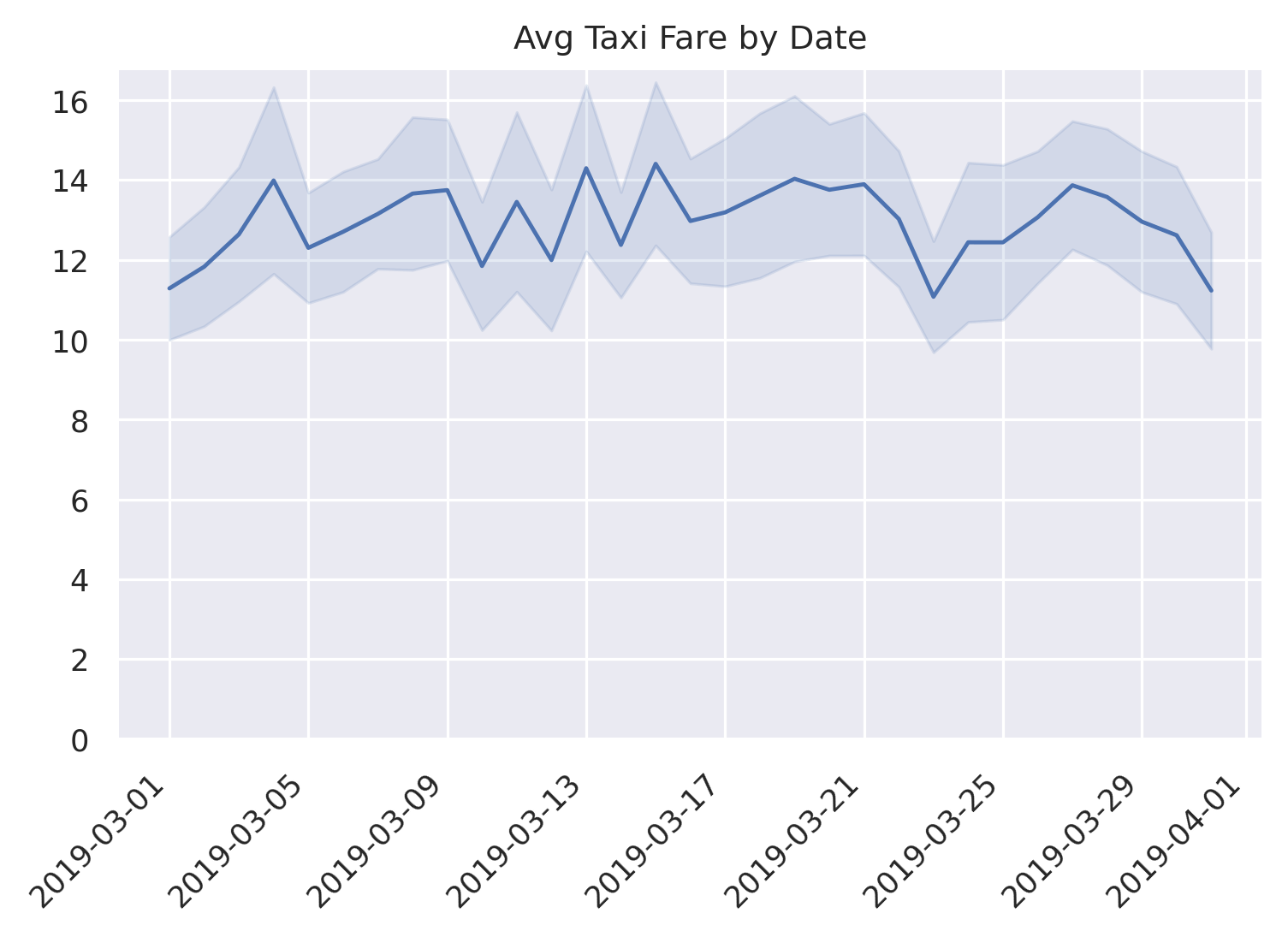## Seaborn

Seaborn is a nice wrapper around Matplotlib so allows for a very similar syntax, but it also comes with some magic if you just give it the raw data.

``````ax = sns.lineplot(data=df, x='pickup_date', y='fare')
# A more verbose but explicit version of the above not relying on defaults:
# ax = sns.lineplot(data=df, x='pickup_date', y='fare', estimator='mean', ci=95,
#                   n_boot=1000)
ax.set_title('Avg Taxi Fare by Date')
ax.set_ylim(0)
ax.figure.autofmt_xdate(rotation=45)
``````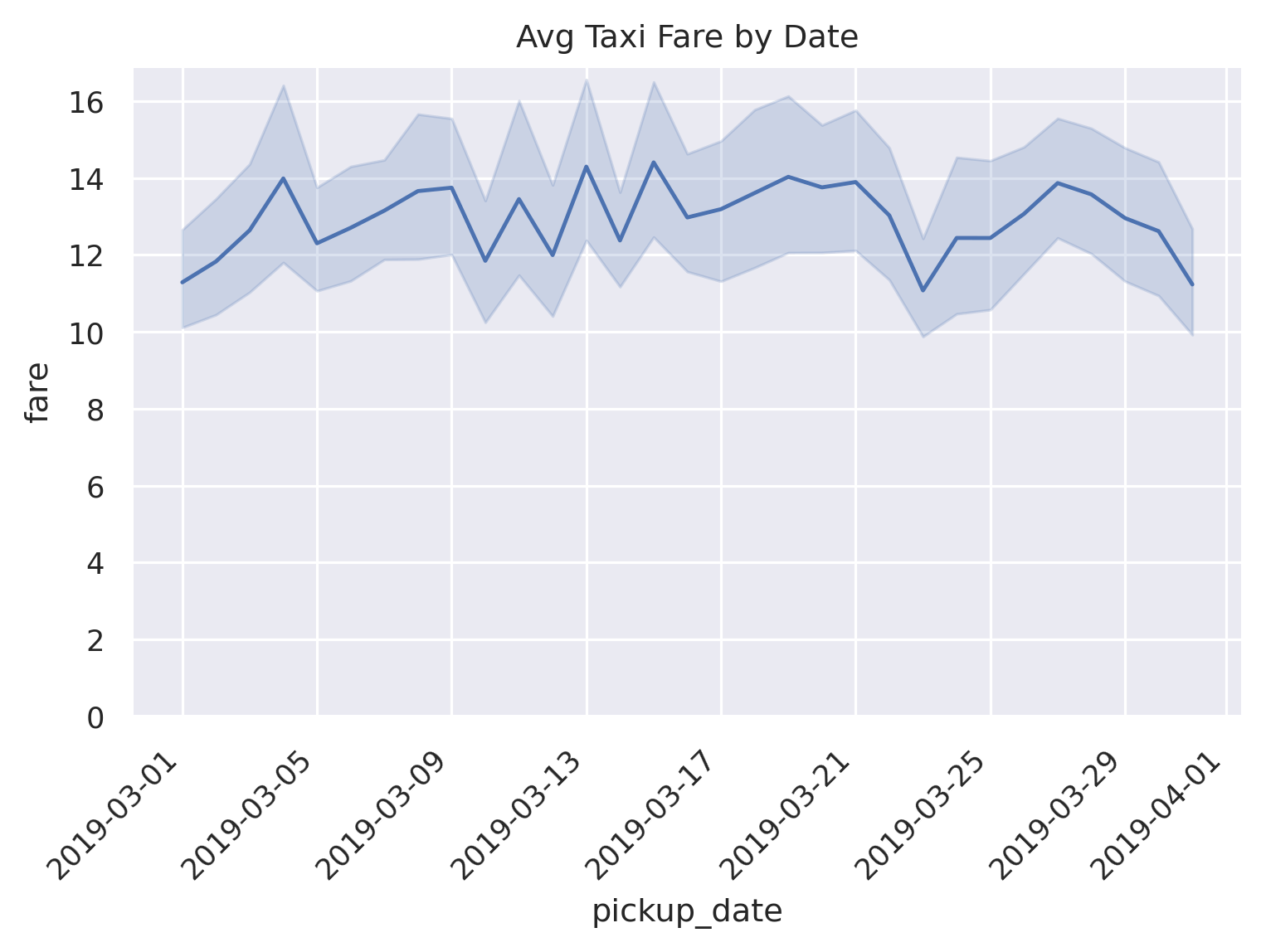By default, if there are more than one observations for each unique `x` value, Seaborn will take the mean of those observations and bootstrap a 95% confidence interval using 1000 iterations. Pretty nice!

We can also do the same thing with the aggregated data, very similarly to Matplotlib.

``````ax = sns.lineplot(data=df_grouped, x='pickup_date', y='mean')
ax.fill_between(
x, df_grouped['ci_lower'], df_grouped['ci_upper'], color='b', alpha=.15)
ax.set_title('Avg Taxi Fare by Date')
ax.set_ylim(0)
ax.figure.autofmt_xdate(rotation=45)
``````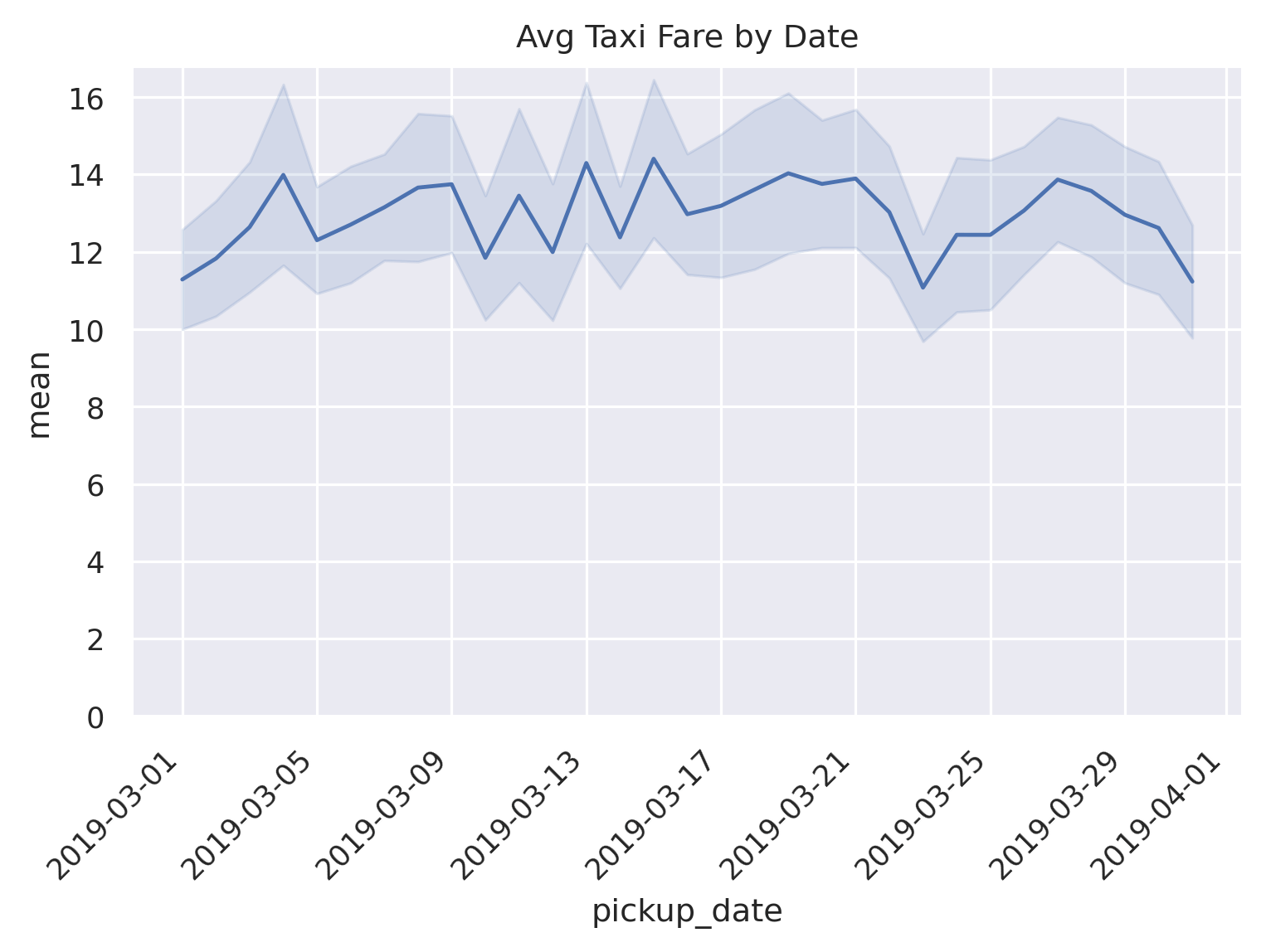## Altair

Altair is similar to Seaborn in that it has some automatated ways of creating and showing a line chart with a confidence interval. The benefit of Altair though is that it's interactive by default (although can be a bit verbose).

We first create the chart using the raw data. Altair allows for aggregate methods in the specification, so `mean(fare)` gets us the average fare for each date. And for the confidence interval, `mark_errorband(extent='ci')` is the magic we need.

``````line = alt.Chart(df).mark_line().encode(
x='pickup_date',
y='mean(fare)'
)

band = alt.Chart(df).mark_errorband(extent='ci').encode(
x=alt.X('pickup_date', title='Avg Fare'),
y=alt.Y('fare', title='Taxi Fare')
)

chart = alt.layer(
band,
line
).properties(
width=600,
height=400,
title='Avg Taxi Fare by Date'
)
chart
``````

Note, this outputs an interactive chart but we just display a static version here since the underlying data for this version (since it uses the raw data) is quite large.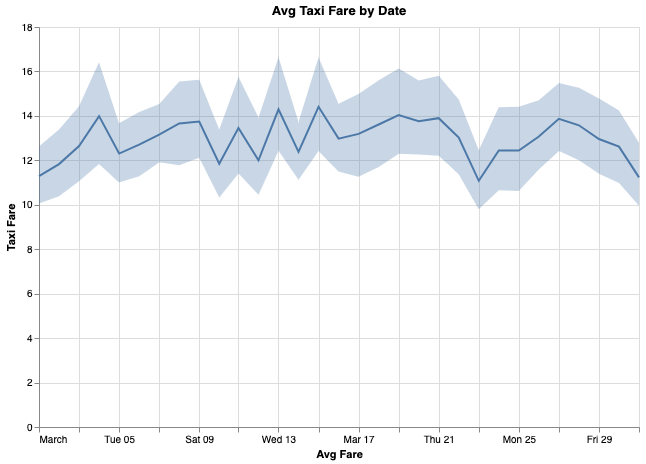We can also easily do this more explicitly using the aggregated data and `mark_area()`. We also add a nice tooltip to display all values on mouseover.

``````line = alt.Chart(df_grouped).mark_line().encode(
x=alt.X('pickup_date:T', title='Pickup Date'),
y=alt.Y('mean:Q', title='Avg Fare')
)

band = alt.Chart(df_grouped).mark_area(
opacity=0.25, color='gray'
).encode(
x='pickup_date:T',
y='ci_lower',
y2='ci_upper',
tooltip=[
'pickup_date:T',
alt.Tooltip('mean', format='.2f'),
alt.Tooltip('ci_lower', format='.2f'),
alt.Tooltip('ci_upper', format='.2f')
]
)

chart = alt.layer(
band,
line
).properties(
width=600,
height=400,
title='Avg Taxi Fare by Date'
)
chart
``````

Below is the embedded output, with tooltip.

Lastly, we go a bit further and use some Altair selection logic to display values alongside a vertical rule, with highlighted points, instead of relying on the tooltip. It's pretty verbose but looks really nice in the end.

``````df_grouped['xlabel'] = 'Avg Fare'
df_grouped['ci_label'] = 'Conf Interval (95%)'
line_color = 'steelblue'
ci_color = 'darkslategray'

line = alt.Chart(df_grouped).mark_line(color=line_color).encode(
x=alt.X('pickup_date:T', title='Pickup Date'),
y=alt.Y('mean:Q', title='Avg Fare')
)

band = alt.Chart(df_grouped).mark_area(
opacity=0.25, color=ci_color
).encode(
x='pickup_date:T',
y='ci_lower:Q',
y2='ci_upper:Q'
)

# Create a selection that chooses the nearest point & selects based on x-value.
nearest = alt.selection(type='single', nearest=True, on='mouseover',
fields=['pickup_date'], empty='none')

# Transparent selectors across the chart. This is what tells us
# the x-value of the cursor.
selectors = alt.Chart(df_grouped).mark_point().encode(
x='pickup_date:T',
opacity=alt.value(0),
nearest
)

# Draw points on the line, and highlight based on selection
points = line.mark_point(color=line_color).encode(
opacity=alt.condition(nearest, alt.value(1), alt.value(0))
)

points2 = band.mark_point(shape='triangle-up').encode(
opacity=alt.condition(nearest, alt.value(1), alt.value(0))
)

points3 = alt.Chart(df_grouped).mark_point(shape='triangle-down').encode(
x='pickup_date:T',
y='ci_upper:Q',
opacity=alt.condition(nearest, alt.value(1), alt.value(0))
)

text = line.mark_text(align='left', dx=5, dy=-5, color=line_color).encode(
text=alt.condition(nearest, 'mean', alt.value(' '), format='.2f')
)

text2 = band.mark_text(align='left', dx=5, dy=5, color=ci_color).encode(
text=alt.condition(nearest, 'ci_lower', alt.value(' '), format='.2f')
)

text3 = alt.Chart(df_grouped).mark_text(
align='left', dx=5, dy=-5, color=ci_color
).encode(
x='pickup_date:T',
y='ci_upper:Q',
text=alt.condition(nearest , 'ci_upper:Q', alt.value(' '), format='.2f'),
)

text4 = alt.Chart(df_grouped).mark_text(align='left', dx=5, dy=10).encode(
x='pickup_date:T',
y=alt.value(0),
text=alt.condition(nearest, 'pickup_date', alt.value(' '))
)

# Draw a rule at the location of the selection
rules = alt.Chart(df_grouped).mark_rule(color='black', strokeDash=[1,1]).encode(
x='pickup_date:T'
).transform_filter(
nearest
)

chart = alt.layer(
band,
line,
selectors,
points,
points2,
points3,
rules,
text,
text2,
text3,
text4
).configure(
# Add padding so the text doesn't get cut off on the right.
padding={"left": 5, "top": 5, "right": 75, "bottom": 5}
).properties(
width=600,
height=400,
title='Avg Taxi Fare by Date'
)

chart
``````

Again, this is very nicely dynamic, hover your mouse over the chart!

## Plotly

Plotly is another interactive visualization library that gives us really nice hover tooltips for free (along with other functionality).

``````fig = go.Figure([
go.Scatter(
name='Avg Fare',
x=df_grouped['pickup_date'],
y=round(df_grouped['mean'], 2),
mode='lines',
line=dict(color='rgb(31, 119, 180)'),
),
go.Scatter(
name='95% CI Upper',
x=df_grouped['pickup_date'],
y=round(df_grouped['ci_upper'], 2),
mode='lines',
marker=dict(color='#444'),
line=dict(width=0),
showlegend=False
),
go.Scatter(
name='95% CI Lower',
x=df_grouped['pickup_date'],
y=round(df_grouped['ci_lower'], 2),
marker=dict(color='#444'),
line=dict(width=0),
mode='lines',
fillcolor='rgba(68, 68, 68, 0.3)',
fill='tonexty',
showlegend=False
)
])
fig.update_layout(
xaxis_title='Pickup Date',
yaxis_title='Avg Fare',
title='Avg Taxi Fare by Date',
hovermode='x'
)
fig.update_yaxes(rangemode='tozero')
``````

Play around with the viz below; pretty slick right?

Another way to do it is to just have one `Scatter` element for the confidence band. You append the upper bound values to the reversed lower bound values and then fill in the area inside of it. The only issue with this method is that getting the nice tooltip for upper/lower bounds isn't possible (or at least isn't easy).

``````fig = go.Figure([
go.Scatter(
name='Avg Fare',
x=df_grouped['pickup_date'],
y=round(df_grouped['mean'], 2),
mode='lines',
line=dict(color='rgb(31, 119, 180)'),
),
go.Scatter(
x=list(df_grouped['pickup_date'])+list(df_grouped['pickup_date'][::-1]), # x, then x reversed
y=list(df_grouped['ci_upper'])+list(df_grouped['ci_lower'][::-1]), # upper, then lower reversed
fill='toself',
fillcolor='rgba(0,100,80,0.2)',
line=dict(color='rgba(255,255,255,0)'),
hoverinfo='skip',
showlegend=False,
name='95% CI'
)
])
fig.update_layout(
xaxis_title='Pickup Date',
yaxis_title='Avg Fare',
title='Avg Taxi Fare by Date',
hovermode='x'
)
fig.update_yaxes(rangemode='tozero')
``````

Again, just saving bandwidth here and embedding the static image here, but this is really an interactive plot.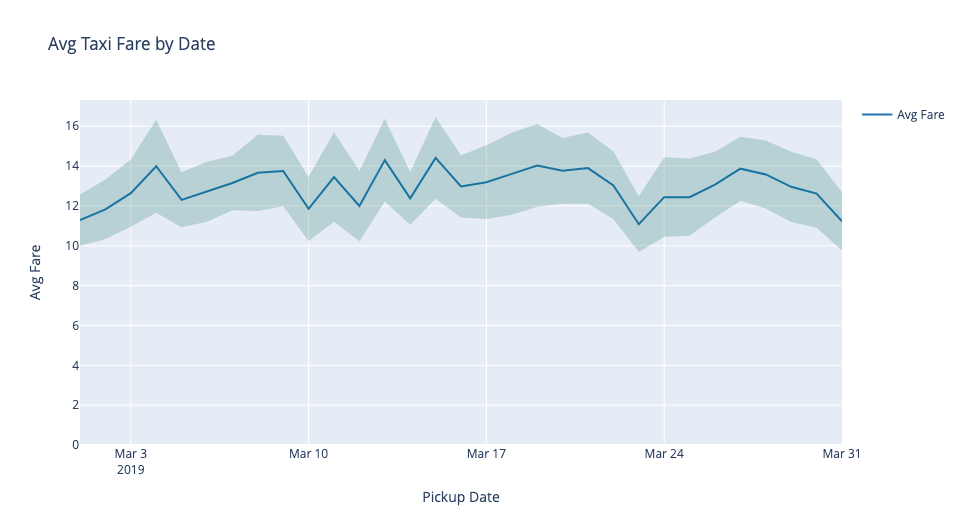And that's how to plot a line chart with a confidence interval using four difference python libraries.